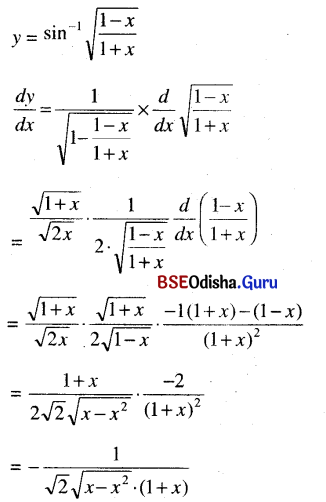# CHSE Odisha Class 12 Math Solutions Chapter 7 Continuity and Differentiability Ex 7(d)

Odisha State Board Elements of Mathematics Class 12 CHSE Odisha Solutions Chapter 7 Continuity and Differentiability Ex 7(d) Textbook Exercise Questions and Answers.

## CHSE Odisha Class 12 Math Solutions Chapter 7 Continuity and Differentiability Exercise 7(d)

Question 1.
Prove the formulae (4) to (7).
Solution:
(4) $$\frac{d}{d x}$$(cos-1 x) = $$\frac{-1}{\sqrt{1-x^2}}$$
Let y = cos-1 x
⇒ x = cos y
⇒ $$\frac{d}{d x}$$ = $$\frac{1}{\left(\frac{d x}{d y}\right)}$$ = $$\frac{1}{-\sin y}$$
But sin y ≥ 0 when
y ∈ [0, π] ( ∵ [0, π] is the principal value branch for cos-1 x)
∴ $$\frac{d y}{d x}$$ = $$\frac{-1}{\sqrt{1-\cos ^2 y}}$$ = $$\frac{-1}{\sqrt{1-x^2}}$$

(5) Let y = tan-1 x
⇒ x = tan y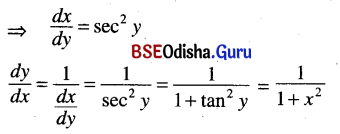(6) Let y = cot-1 x
⇒ x = cot y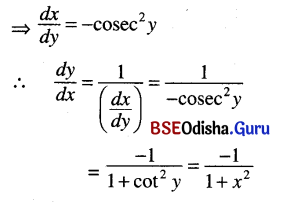(7) Let y = cosec-1 x
⇒ x = cosec y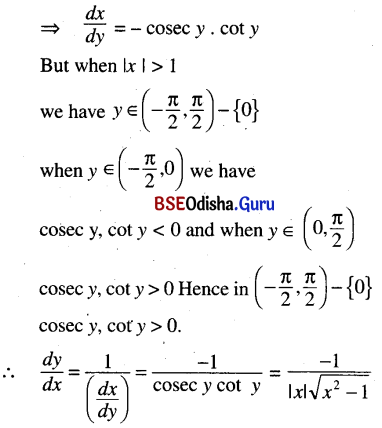Question 2.
Find derivatives of the following functions.
sin-1 2x
Solution:
y = sin-1 2x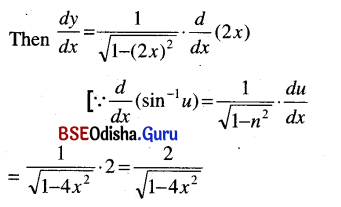Question 3.
cot-1 √x
Solution:
cot-1 √x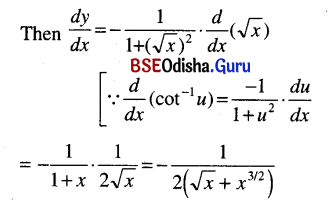Question 4.
sec-1 (2x + 1)
Solution:
y = sec-1 (2x + 1)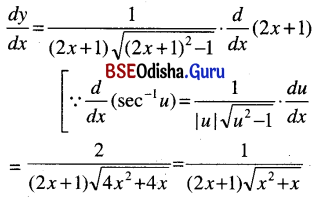Question 5.
cos-1 $$\sqrt{\frac{1+x}{2}}$$
Solution: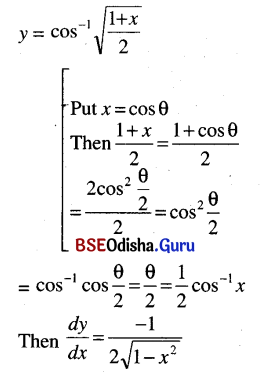Question 6.
cos-1 $$\left(\frac{x-\frac{1}{x}}{x+\frac{1}{x}}\right)$$
Solution: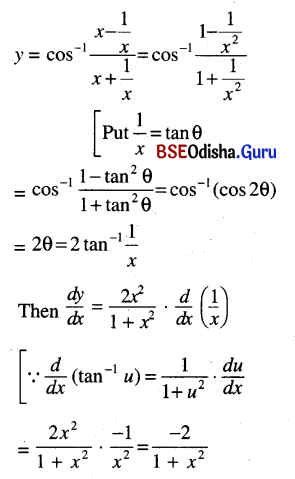Question 7.
tan-1 (cos √x)
Solution:
y = tan-1 (cos √x)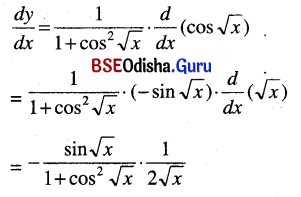Question 8.
x2 cosec-1 $$\left(\frac{1}{\ln x}\right)$$
Solution: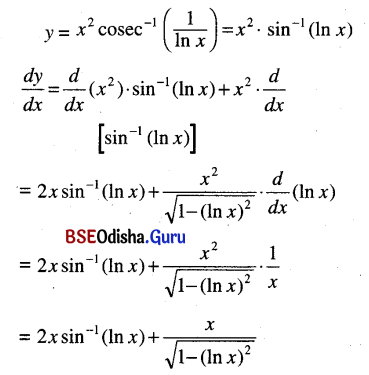Question 9.
cot-1 $$\frac{\sqrt{1-x^2}}{x}$$
Solution: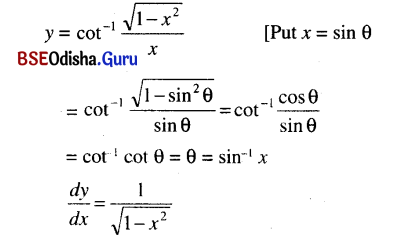Question 10.
(x sin-1 x)15
Solution:
y = (x sin-1 x)15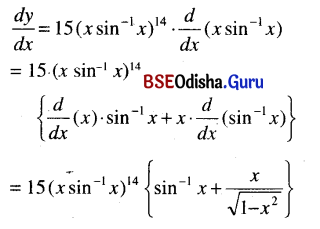Question 11.
sin-1 $$\sqrt{\frac{1-x}{1+x}}$$
Solution: